### Two-node 3-dimensional spring (SPRINGA)

This is a spring element defined between two nodes (Figure 79). The force needed in node 2 to extend the spring with original length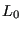to a final length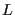is given by: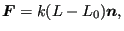(18)

where k is the spring stiffness and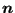is a unit vector pointing from node 1 to node 2. The force in node 1 is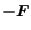. This formula applies if the spring stiffness is constant. It is defined using the *SPRING keyword card. Alternatively, a nonlinear spring can be defined by providing a graph of the force versus the elongation. In calculations in which NLGEOM is active (nonlinear geometric calculations) the motion of nodes 1 and 2 induces a change of.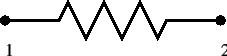The two-node three-dimensional spring element is considered as a genuine three-dimensional element. Consequently, if it is connected to a 2D element with special restraints on the third direction (plane stress, plane strain or axisymmetric elements) the user has to take care that the third dimension does not induce rigid body motions in the spring nodes. An example of how to restrain the spring is given in test example spring4.

Note that a spring under compression, if not properly restrained, may change its direction by 180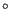, leading to unexpected results. Furthermore, for nonlinear springs, it does not make sense to extend the force-elongation curve to negative elongation values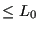.